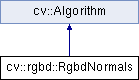OpenCV  3.0.0-dev Open Source Computer Vision
cv::rgbd::RgbdNormals Class Reference

#include "rgbd.hpp"

Inheritance diagram for cv::rgbd::RgbdNormals:## Public Types

enum  RGBD_NORMALS_METHOD {
RGBD_NORMALS_METHOD_FALS,
RGBD_NORMALS_METHOD_LINEMOD,
RGBD_NORMALS_METHOD_SRI
}

## Public Member Functions

RgbdNormals ()

RgbdNormals (int rows, int cols, int depth, InputArray K, int window_size=5, int method=RGBD_NORMALS_METHOD_FALS)

~RgbdNormals ()

int getCols () const

int getDepth () const

cv::Mat getK () const

int getMethod () const

int getRows () const

int getWindowSize () const

void initialize () const

void operator() (InputArray points, OutputArray normals) const

void setCols (int val)

void setDepth (int val)

void setK (const cv::Mat &val)

void setMethod (int val)

void setRows (int val)

void setWindowSize (int val)Public Member Functions inherited from cv::Algorithm
Algorithm ()

virtual ~Algorithm ()

virtual void clear ()
Clears the algorithm state. More...

virtual bool empty () const
Returns true if the Algorithm is empty (e.g. in the very beginning or after unsuccessful read. More...

virtual String getDefaultName () const

virtual void read (const FileNode &fn)
Reads algorithm parameters from a file storage. More...

virtual void save (const String &filename) const

virtual void write (FileStorage &fs) const
Stores algorithm parameters in a file storage. More...

## Protected Member Functions

void initialize_normals_impl (int rows, int cols, int depth, const Mat &K, int window_size, int method) const

## Protected Attributes

int cols_

int depth_

Mat K_

int method_

void * rgbd_normals_impl_

int rows_

int window_size_Static Public Member Functions inherited from cv::Algorithm
template<typename _Tp >
static Ptr< _Tp > load (const String &filename, const String &objname=String())
Loads algorithm from the file. More...

template<typename _Tp >
static Ptr< _Tp > loadFromString (const String &strModel, const String &objname=String())
Loads algorithm from a String. More...

template<typename _Tp >
static Ptr< _Tp > read (const FileNode &fn)
Reads algorithm from the file node. More...

## Detailed Description

Object that can compute the normals in an image. It is an object as it can cache data for speed efficiency The implemented methods are either:

• FALS (the fastest) and SRI from Fast and Accurate Computation of Surface Normals from Range Images by H. Badino, D. Huber, Y. Park and T. Kanade
• the normals with bilateral filtering on a depth image from Gradient Response Maps for Real-Time Detection of Texture-Less Objects by S. Hinterstoisser, C. Cagniart, S. Ilic, P. Sturm, N. Navab, P. Fua, and V. Lepetit

## Constructor & Destructor Documentation

 cv::rgbd::RgbdNormals::RgbdNormals ( )
inline
 cv::rgbd::RgbdNormals::RgbdNormals ( int rows, int cols, int depth, InputArray K, int window_size = 5, int method = RGBD_NORMALS_METHOD_FALS )

Constructor

Parameters
 rows the number of rows of the depth image normals will be computed on cols the number of cols of the depth image normals will be computed on depth the depth of the normals (only CV_32F or CV_64F for FALS and SRI, CV_16U for LINEMOD) K the calibration matrix to use window_size the window size to compute the normals: can only be 1,3,5 or 7 method one of the methods to use: RGBD_NORMALS_METHOD_SRI, RGBD_NORMALS_METHOD_FALS
 cv::rgbd::RgbdNormals::~RgbdNormals ( )

## Member Function Documentation

 int cv::rgbd::RgbdNormals::getCols ( ) const
inline
 int cv::rgbd::RgbdNormals::getDepth ( ) const
inline
 cv::Mat cv::rgbd::RgbdNormals::getK ( ) const
inline
 int cv::rgbd::RgbdNormals::getMethod ( ) const
inline
 int cv::rgbd::RgbdNormals::getRows ( ) const
inline
 int cv::rgbd::RgbdNormals::getWindowSize ( ) const
inline
 void cv::rgbd::RgbdNormals::initialize ( ) const

Initializes some data that is cached for later computation If that function is not called, it will be called the first time normals are computed

 void cv::rgbd::RgbdNormals::initialize_normals_impl ( int rows, int cols, int depth, const Mat & K, int window_size, int method ) const
protected
 void cv::rgbd::RgbdNormals::operator() ( InputArray points, OutputArray normals ) const

Given a set of 3d points in a depth image, compute the normals at each point.

Parameters
 points a rows x cols x 3 matrix of CV_32F/CV64F or a rows x cols x 1 CV_U16S normals a rows x cols x 3 matrix
 void cv::rgbd::RgbdNormals::setCols ( int val )
inline
 void cv::rgbd::RgbdNormals::setDepth ( int val )
inline
 void cv::rgbd::RgbdNormals::setK ( const cv::Mat & val )
inline
 void cv::rgbd::RgbdNormals::setMethod ( int val )
inline
 void cv::rgbd::RgbdNormals::setRows ( int val )
inline
 void cv::rgbd::RgbdNormals::setWindowSize ( int val )
inline

## Member Data Documentation

 int cv::rgbd::RgbdNormals::cols_
protected
 int cv::rgbd::RgbdNormals::depth_
protected
 Mat cv::rgbd::RgbdNormals::K_
protected
 int cv::rgbd::RgbdNormals::method_
protected
 void* cv::rgbd::RgbdNormals::rgbd_normals_impl_
mutableprotected
 int cv::rgbd::RgbdNormals::rows_
protected
 int cv::rgbd::RgbdNormals::window_size_
protected

The documentation for this class was generated from the following file:
• /build/master-contrib_docs-mac/opencv_contrib/modules/rgbd/include/opencv2/rgbd.hpp